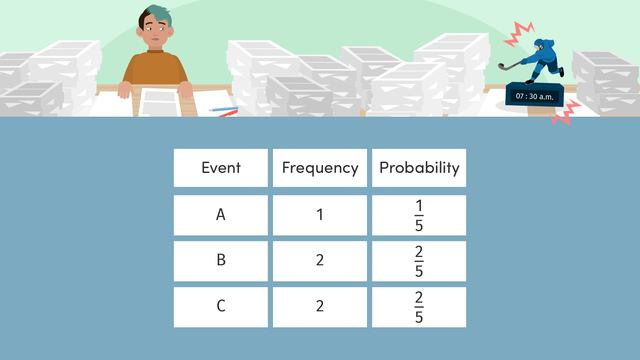# Developing a Probability ModelRating

Be the first to give a rating!
The authorsTeam Digital
Developing a Probability Model
CCSS.MATH.CONTENT.7.SP.C.7

## Basics on the topicDeveloping a Probability Model

Developing a Probability Model Students can make a probability model (table) with given information, and they can interpret a probability model.

### TranscriptDeveloping a Probability Model

Kai, who is captain of the Arctic Wolves, is gearing up for a season opener against the Glacier Gliders. He is looking back on last year's stats to make predictions on how many goals each team will score in the game. Kai decides that Developing a Probability Model is the best way to display the data. This model will display the frequencies or outcomes of an event, and in this case the number of goals scored in a game. A probability model can be in the form of a table to display the events, frequencies, and probabilities. This table shows all the possible outcomes of an event, and therefore the probabilities all need to add to one whole. Kai begins to fill in the table with his team's data, The Arctic Wolves! He looks at the past season's final scores and records the goal outcomes. The frequency column shows how many times the team scored that amount of goals in a game. Remember, the probability of an event measures the likelihood it will occur. First, find the total number of games, twenty. During last season, there were two games with no goals, and the probability would be two out of twenty. This probability can simplify to a one out of ten chance of not scoring at all. The next probability is five out of twenty, and simplifies to one-fourth. The last rows would be nine out of twenty, one out of twenty, and three out of twenty none of which need to be simplified. Let's check for discrepancies and find the sum of our probabilities. These values add to one, to represent the whole season, therefore our table is accurate. Kai also wants to analyze the Glacier Glider's stats over their season, but we need to help him complete the missing row! To find the last row, we need to remember that there are twenty total games in a season. Also, for the table to have no discrepancies, the probabilities need to have a sum of one. The common denominator for the probabilities is twenty. When we add them we have a sum of nineteen over twenty. The difference between twenty and nineteen is one so the probability that they scored four or more goals was one game out of twenty. Let's answer a few questions about our two probability models. Based on last year's stats, what was the probability for the Arctic Wolves that the team scored two or more goals? The sum of these probabilities would be a thirteen out of twenty chance that the Arctic Wolves would score at least two goals in a game. Based on last year's stats, which team had a higher probability of having a game that they scored three or more goals in? Both teams are predicted to have the same one-in-five chance that they will have a game where they score three or more goals. It looks like this match up between the Arctic Wolves and the Glacier Gliders is going to be a close one! To summarize, developing a probability model is a way to display the frequency and probabilities of an event occurring. Remember, the probabilities must always add to one to prove no discrepancies. Creating this type of model can be helpful when needing to make predictions for the future. Good thing Kai set an alarm, otherwise he would have probably missed the first game!